Скачать презентацию Chapter 18 Real Estate Finance Tools Present Value

• Количество слайдов: 23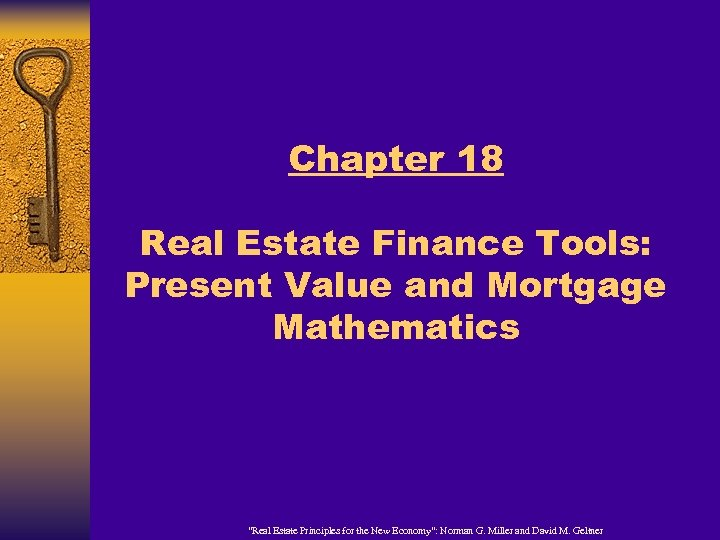Chapter 18 Real Estate Finance Tools: Present Value and Mortgage Mathematics “Real Estate Principles for the New Economy”: Norman G. Miller and David M. Geltner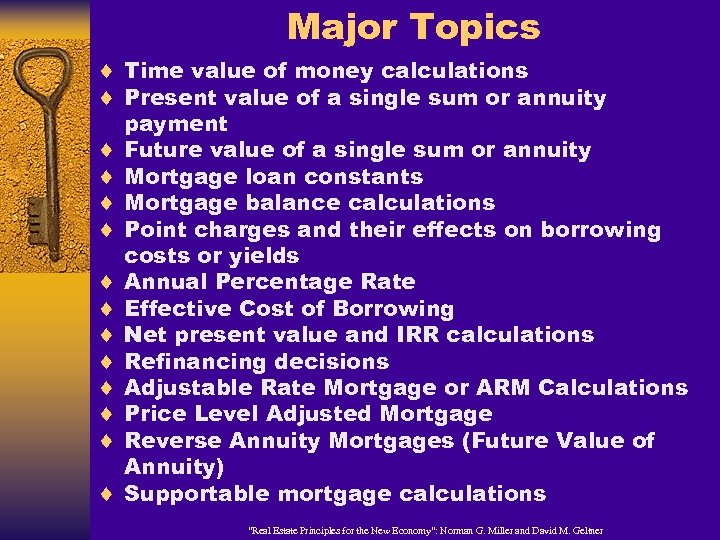Major Topics ¨ Time value of money calculations ¨ Present value of a single sum or annuity ¨ ¨ ¨ payment Future value of a single sum or annuity Mortgage loan constants Mortgage balance calculations Point charges and their effects on borrowing costs or yields Annual Percentage Rate Effective Cost of Borrowing Net present value and IRR calculations Refinancing decisions Adjustable Rate Mortgage or ARM Calculations Price Level Adjusted Mortgage Reverse Annuity Mortgages (Future Value of Annuity) Supportable mortgage calculations “Real Estate Principles for the New Economy”: Norman G. Miller and David M. Geltner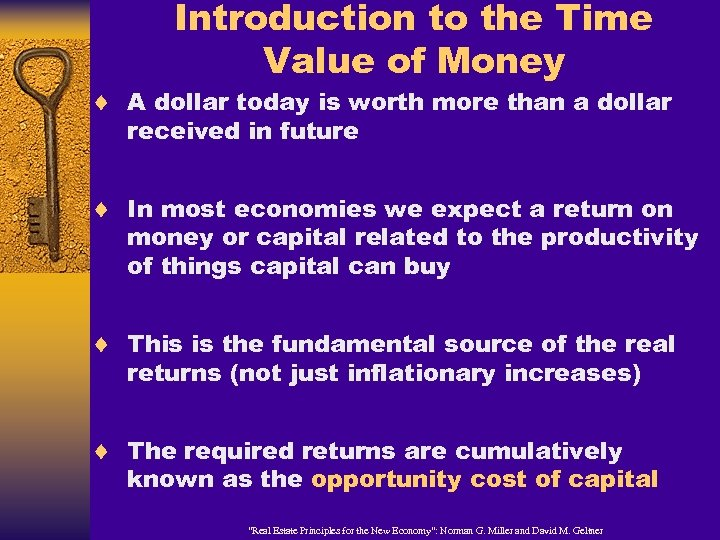Introduction to the Time Value of Money ¨ A dollar today is worth more than a dollar received in future ¨ In most economies we expect a return on money or capital related to the productivity of things capital can buy ¨ This is the fundamental source of the real returns (not just inflationary increases) ¨ The required returns are cumulatively known as the opportunity cost of capital “Real Estate Principles for the New Economy”: Norman G. Miller and David M. Geltner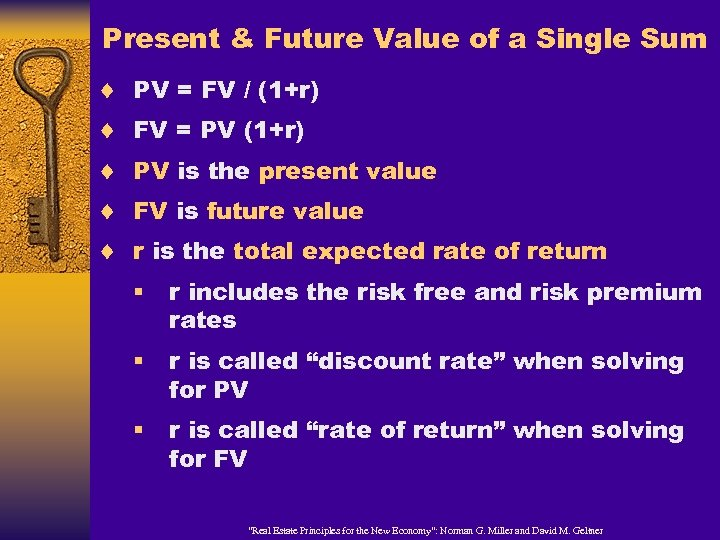Present & Future Value of a Single Sum ¨ ¨ ¨ PV = FV / (1+r) FV = PV (1+r) PV is the present value FV is future value r is the total expected rate of return § r includes the risk free and risk premium rates § r is called “discount rate” when solving for PV § r is called “rate of return” when solving for FV “Real Estate Principles for the New Economy”: Norman G. Miller and David M. Geltner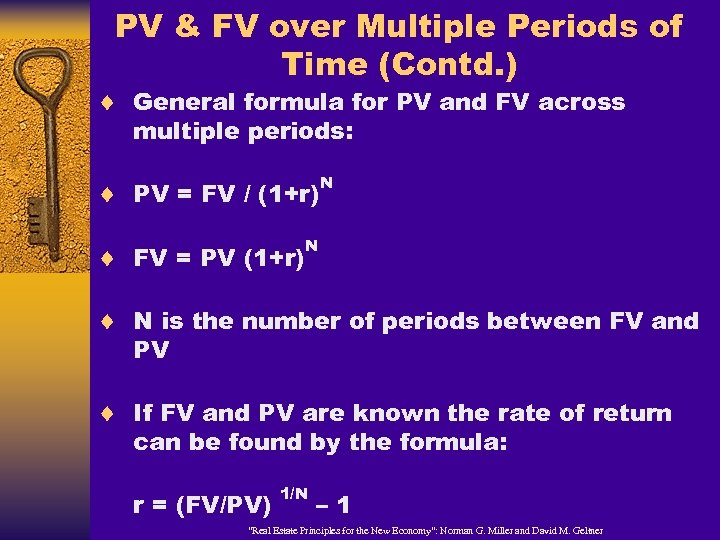PV & FV over Multiple Periods of Time (Contd. ) ¨ General formula for PV and FV across multiple periods: ¨ PV = FV / (1+r) ¨ FV = PV (1+r) N N ¨ N is the number of periods between FV and PV ¨ If FV and PV are known the rate of return can be found by the formula: r = (FV/PV) 1/N – 1 “Real Estate Principles for the New Economy”: Norman G. Miller and David M. Geltner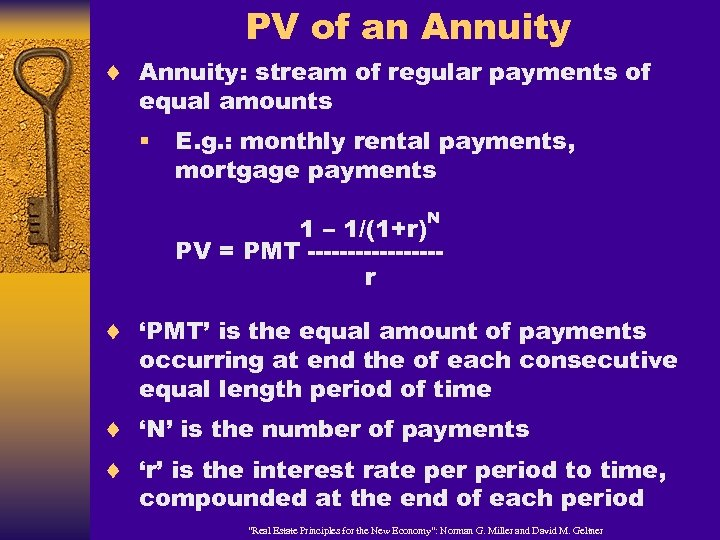PV of an Annuity ¨ Annuity: stream of regular payments of equal amounts § E. g. : monthly rental payments, mortgage payments N 1 – 1/(1+r) PV = PMT --------r ¨ ‘PMT’ is the equal amount of payments occurring at end the of each consecutive equal length period of time ¨ ‘N’ is the number of payments ¨ ‘r’ is the interest rate period to time, compounded at the end of each period “Real Estate Principles for the New Economy”: Norman G. Miller and David M. Geltner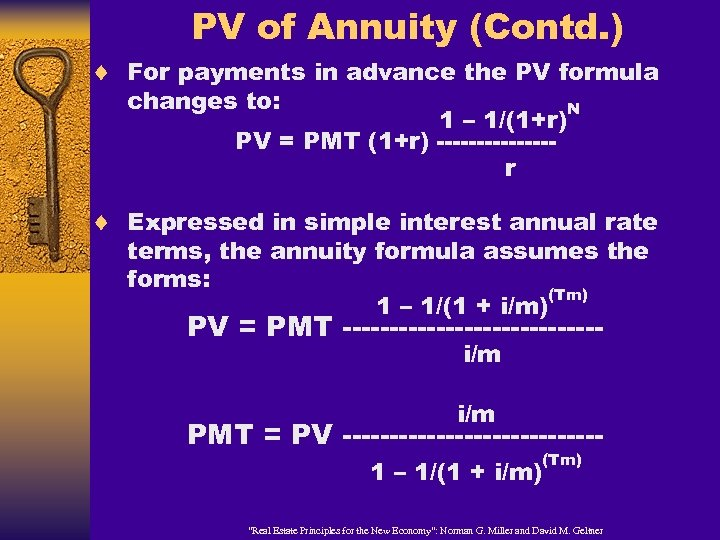PV of Annuity (Contd. ) ¨ For payments in advance the PV formula changes to: 1 – 1/(1+r) PV = PMT (1+r) -------r N ¨ Expressed in simple interest annual rate terms, the annuity formula assumes the forms: (Tm) 1 – 1/(1 + i/m) PV = PMT --------------i/m PMT = PV --------------1 – 1/(1 + i/m) (Tm) “Real Estate Principles for the New Economy”: Norman G. Miller and David M. Geltner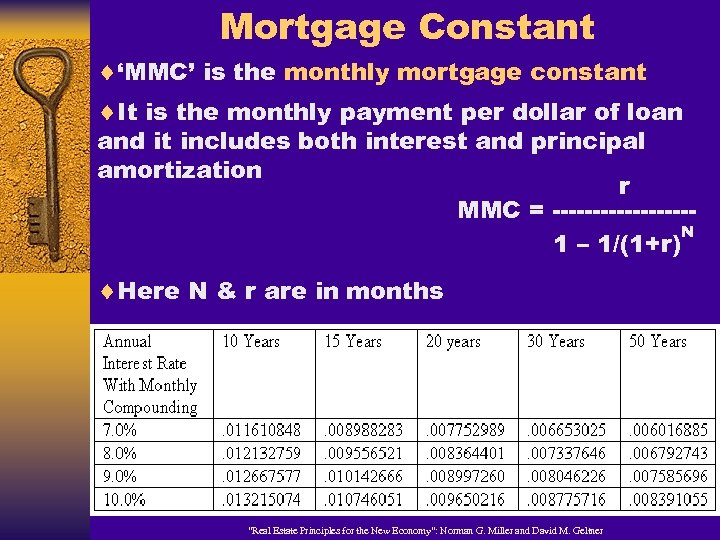Mortgage Constant ¨‘MMC’ is the monthly mortgage constant ¨It is the monthly payment per dollar of loan and it includes both interest and principal amortization r MMC = ---------N 1 – 1/(1+r) ¨Here N & r are in months “Real Estate Principles for the New Economy”: Norman G. Miller and David M. Geltner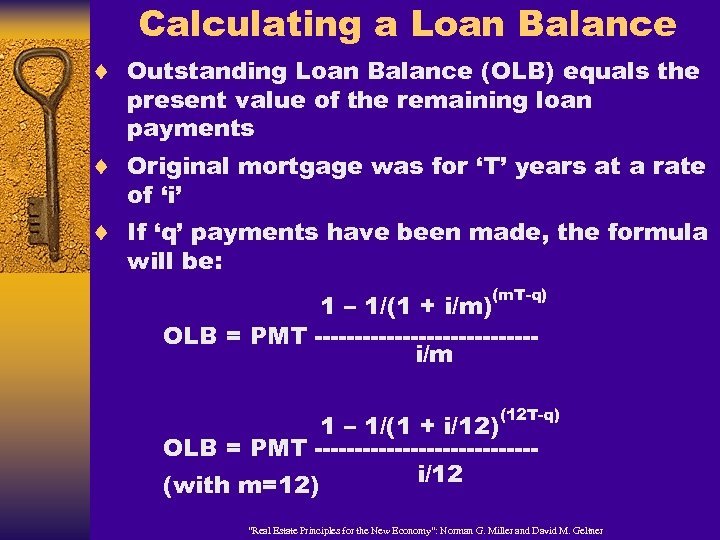Calculating a Loan Balance ¨ Outstanding Loan Balance (OLB) equals the present value of the remaining loan payments ¨ Original mortgage was for ‘T’ years at a rate of ‘i’ ¨ If ‘q’ payments have been made, the formula will be: (m. T-q) 1 – 1/(1 + i/m) OLB = PMT --------------i/m (12 T-q) 1 – 1/(1 + i/12) OLB = PMT --------------i/12 (with m=12) “Real Estate Principles for the New Economy”: Norman G. Miller and David M. Geltner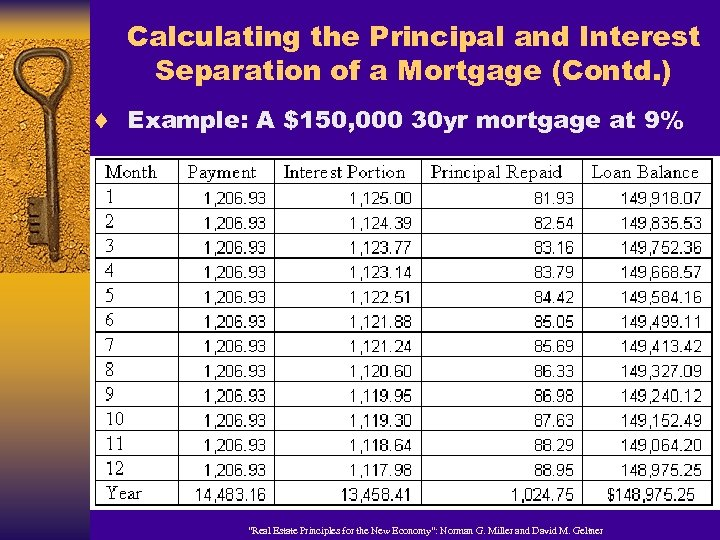Calculating the Principal and Interest Separation of a Mortgage (Contd. ) ¨ Example: A \$150, 000 30 yr mortgage at 9% “Real Estate Principles for the New Economy”: Norman G. Miller and David M. Geltner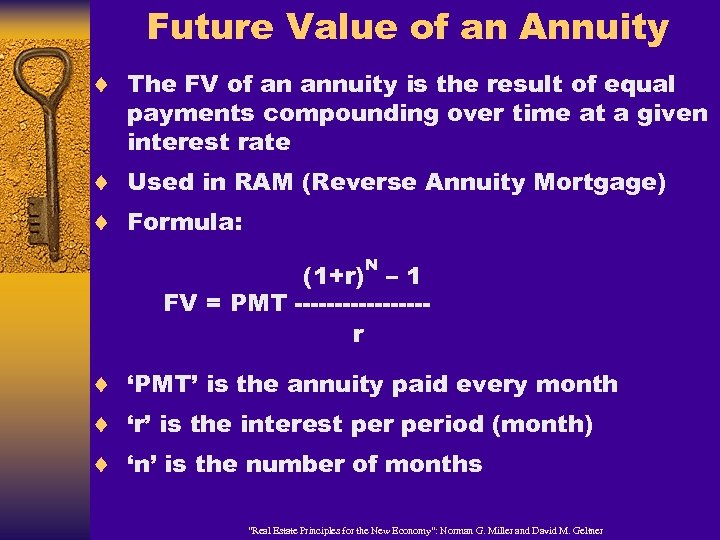Future Value of an Annuity ¨ The FV of an annuity is the result of equal payments compounding over time at a given interest rate ¨ Used in RAM (Reverse Annuity Mortgage) ¨ Formula: N (1+r) – 1 FV = PMT --------r ¨ ‘PMT’ is the annuity paid every month ¨ ‘r’ is the interest period (month) ¨ ‘n’ is the number of months “Real Estate Principles for the New Economy”: Norman G. Miller and David M. Geltner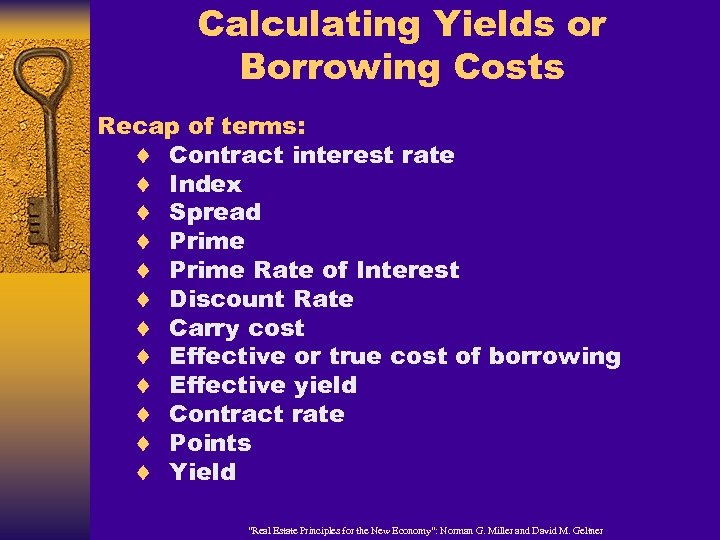Calculating Yields or Borrowing Costs Recap of terms: ¨ Contract interest rate ¨ Index ¨ Spread ¨ Prime Rate of Interest ¨ Discount Rate ¨ Carry cost ¨ Effective or true cost of borrowing ¨ Effective yield ¨ Contract rate ¨ Points ¨ Yield “Real Estate Principles for the New Economy”: Norman G. Miller and David M. Geltner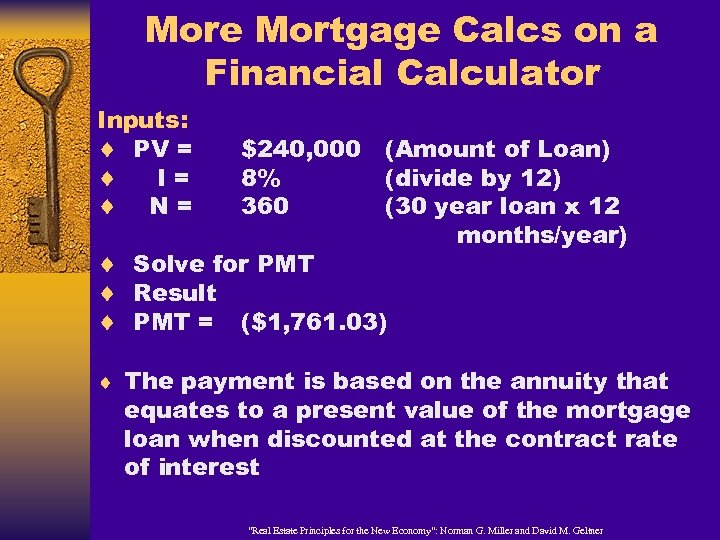More Mortgage Calcs on a Financial Calculator Inputs: ¨ PV = ¨ I= ¨ N= \$240, 000 8% 360 (Amount of Loan) (divide by 12) (30 year loan x 12 months/year) ¨ Solve for PMT ¨ Result ¨ PMT = (\$1, 761. 03) ¨ The payment is based on the annuity that equates to a present value of the mortgage loan when discounted at the contract rate of interest “Real Estate Principles for the New Economy”: Norman G. Miller and David M. Geltner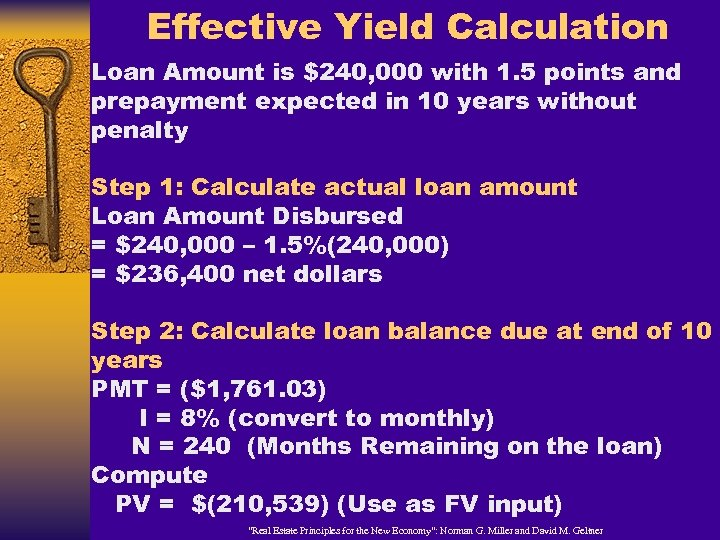Effective Yield Calculation Loan Amount is \$240, 000 with 1. 5 points and prepayment expected in 10 years without penalty Step 1: Calculate actual loan amount Loan Amount Disbursed = \$240, 000 – 1. 5%(240, 000) = \$236, 400 net dollars Step 2: Calculate loan balance due at end of 10 years PMT = (\$1, 761. 03) I = 8% (convert to monthly) N = 240 (Months Remaining on the loan) Compute PV = \$(210, 539) (Use as FV input) “Real Estate Principles for the New Economy”: Norman G. Miller and David M. Geltner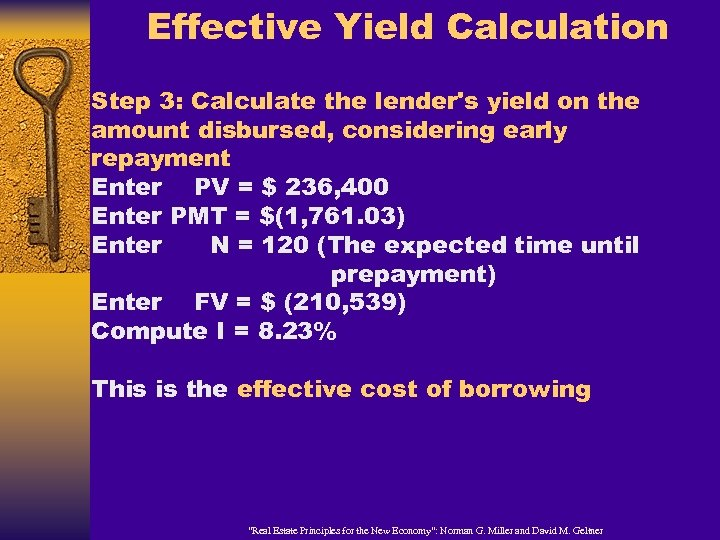Effective Yield Calculation Step 3: Calculate the lender's yield on the amount disbursed, considering early repayment Enter PV = \$ 236, 400 Enter PMT = \$(1, 761. 03) Enter N = 120 (The expected time until prepayment) Enter FV = \$ (210, 539) Compute I = 8. 23% This is the effective cost of borrowing “Real Estate Principles for the New Economy”: Norman G. Miller and David M. Geltner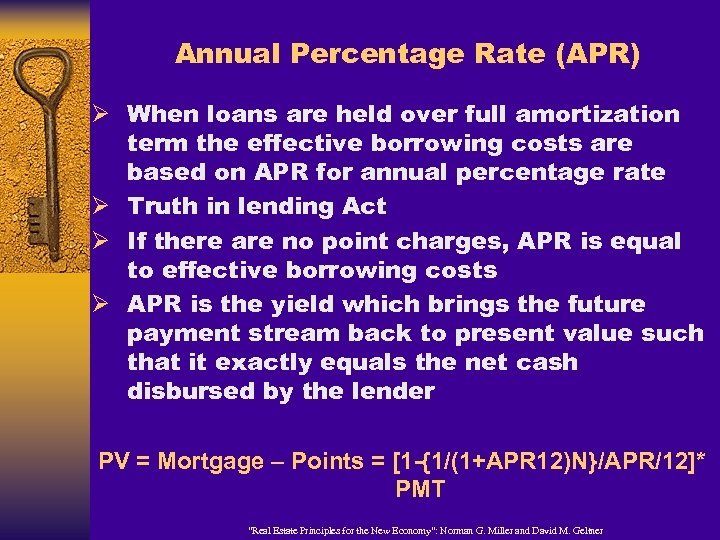Annual Percentage Rate (APR) Ø When loans are held over full amortization term the effective borrowing costs are based on APR for annual percentage rate Ø Truth in lending Act Ø If there are no point charges, APR is equal to effective borrowing costs Ø APR is the yield which brings the future payment stream back to present value such that it exactly equals the net cash disbursed by the lender PV = Mortgage – Points = [1 -{1/(1+APR 12)N}/APR/12]* PMT “Real Estate Principles for the New Economy”: Norman G. Miller and David M. Geltner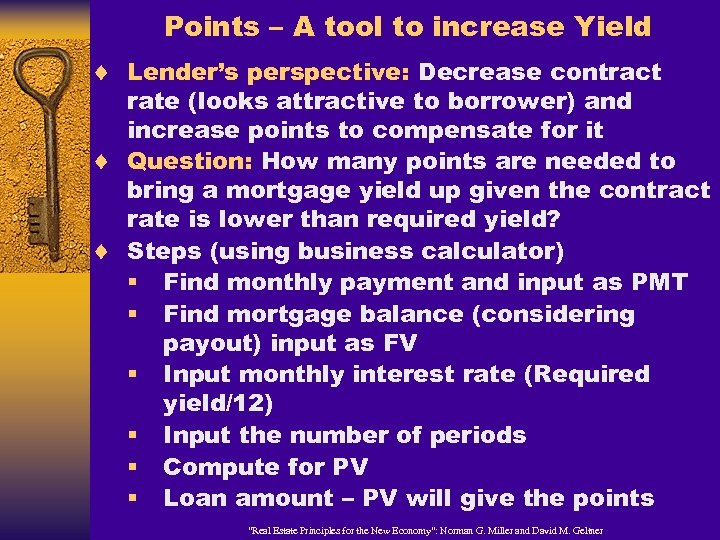Points – A tool to increase Yield ¨ Lender’s perspective: Decrease contract rate (looks attractive to borrower) and increase points to compensate for it ¨ Question: How many points are needed to bring a mortgage yield up given the contract rate is lower than required yield? ¨ Steps (using business calculator) § Find monthly payment and input as PMT § Find mortgage balance (considering payout) input as FV § Input monthly interest rate (Required yield/12) § Input the number of periods § Compute for PV § Loan amount – PV will give the points “Real Estate Principles for the New Economy”: Norman G. Miller and David M. Geltner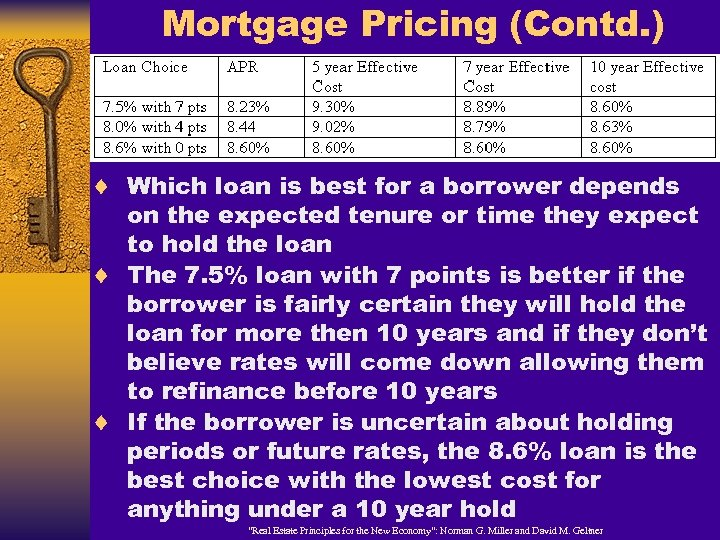Mortgage Pricing (Contd. ) ¨ Which loan is best for a borrower depends on the expected tenure or time they expect to hold the loan ¨ The 7. 5% loan with 7 points is better if the borrower is fairly certain they will hold the loan for more then 10 years and if they don’t believe rates will come down allowing them to refinance before 10 years ¨ If the borrower is uncertain about holding periods or future rates, the 8. 6% loan is the best choice with the lowest cost for anything under a 10 year hold “Real Estate Principles for the New Economy”: Norman G. Miller and David M. Geltner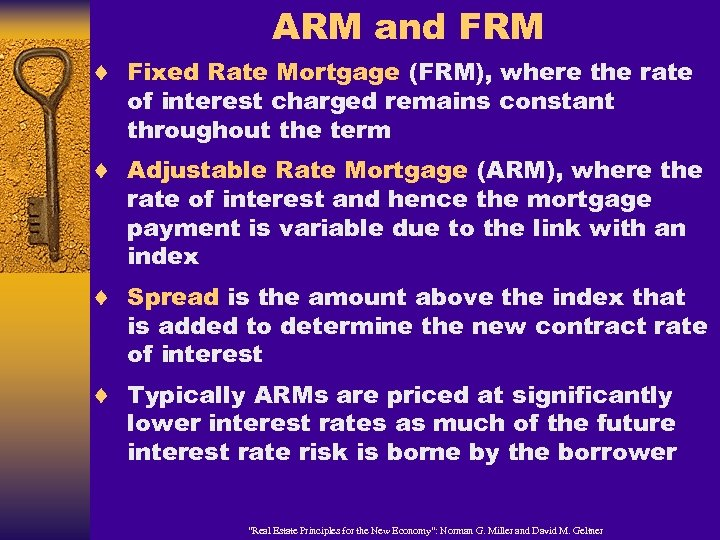ARM and FRM ¨ Fixed Rate Mortgage (FRM), where the rate of interest charged remains constant throughout the term ¨ Adjustable Rate Mortgage (ARM), where the rate of interest and hence the mortgage payment is variable due to the link with an index ¨ Spread is the amount above the index that is added to determine the new contract rate of interest ¨ Typically ARMs are priced at significantly lower interest rates as much of the future interest rate risk is borne by the borrower “Real Estate Principles for the New Economy”: Norman G. Miller and David M. Geltner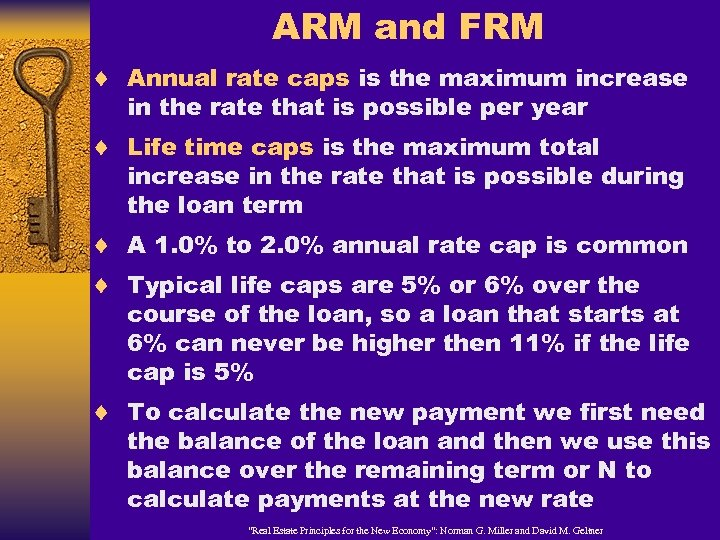ARM and FRM ¨ Annual rate caps is the maximum increase in the rate that is possible per year ¨ Life time caps is the maximum total increase in the rate that is possible during the loan term ¨ A 1. 0% to 2. 0% annual rate cap is common ¨ Typical life caps are 5% or 6% over the course of the loan, so a loan that starts at 6% can never be higher then 11% if the life cap is 5% ¨ To calculate the new payment we first need the balance of the loan and then we use this balance over the remaining term or N to calculate payments at the new rate “Real Estate Principles for the New Economy”: Norman G. Miller and David M. Geltner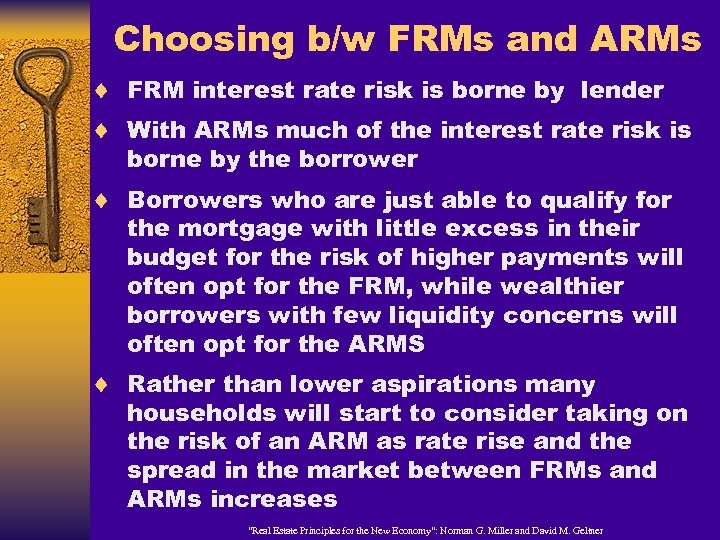Choosing b/w FRMs and ARMs ¨ FRM interest rate risk is borne by lender ¨ With ARMs much of the interest rate risk is borne by the borrower ¨ Borrowers who are just able to qualify for the mortgage with little excess in their budget for the risk of higher payments will often opt for the FRM, while wealthier borrowers with few liquidity concerns will often opt for the ARMS ¨ Rather than lower aspirations many households will start to consider taking on the risk of an ARM as rate rise and the spread in the market between FRMs and ARMs increases “Real Estate Principles for the New Economy”: Norman G. Miller and David M. Geltner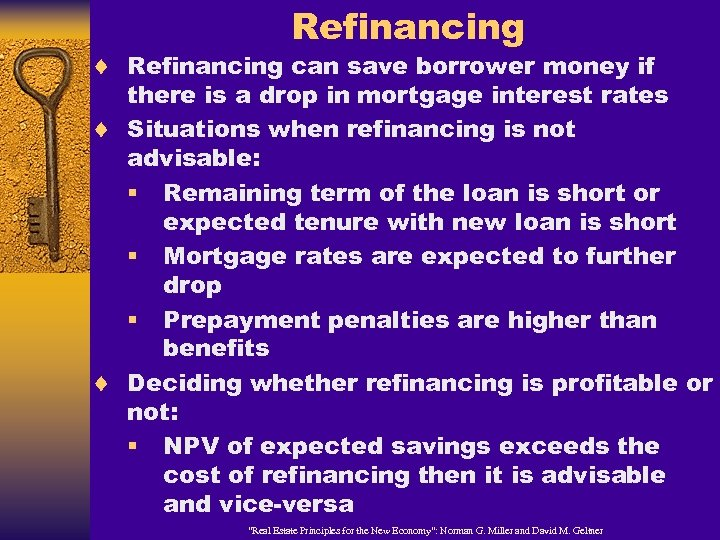Refinancing ¨ Refinancing can save borrower money if there is a drop in mortgage interest rates ¨ Situations when refinancing is not advisable: § Remaining term of the loan is short or expected tenure with new loan is short § Mortgage rates are expected to further drop § Prepayment penalties are higher than benefits ¨ Deciding whether refinancing is profitable or not: § NPV of expected savings exceeds the cost of refinancing then it is advisable and vice-versa “Real Estate Principles for the New Economy”: Norman G. Miller and David M. GeltnerEND “Real Estate Principles for the New Economy”: Norman G. Miller and David M. Geltner